# ACCRINTM Function

Calculates the accrued interest for a security that pays interest on maturity

## What is the ACCRINTM Function?

The ACCRINTM Function is an Excel Financial function. ACCRINTM was introduced in MS Excel 2007 and hence not available in earlier versions.

When we invest in an interest-paying security, we will receive interest payments that are paid either at once or periodically. The function will calculate the accrued interest for a security that pays interest on maturity.

MS Excel provides two functions for calculating accrued interest: ACCRINT and ACCRINTM. ACCRINT helps calculate the interest that is paid by the issuer periodically. On the other hand, ACCRINTM calculates the interest that is paid at maturity, or the lump sum interest when a security expires/matures.

### Formula

=ACCRINTM(issue, settlement, rate, par, [basis])

The ACCRINTM function uses the following arguments:

1. Issue (required argument) – This is the security’s issue date. If it is not an integer, it will be truncated.
2. Settlement (required argument) – The security’s maturity date, which is when the security expires.
3. Rate (required argument) – This is the security’s annual coupon rate.
4. Par (required argument) – The security’s par value. If omitted, then ACCRINTM function will take the par value as \$1,000.
5. Basis (optional argument) – This is the kind of day count that is used for calculating the interest on a given security. If we omit the basis argument, it is set to 0. Basis can be any of the following values:

BasisDay Count basis
0 or omittedUS(NASD) 30/360
1Actual/actual
2Actual/360
3Actual/365
4European 30/360

### How to use the ACCRINTM Function in Excel?

To understand the uses of the ACCRINTM function, let’s consider a few examples:

#### Example 1

Suppose we are given the following data: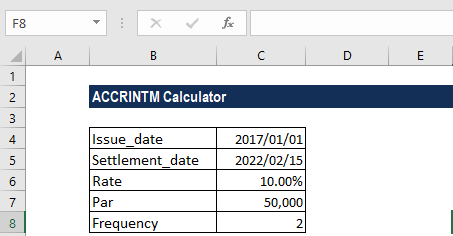The formula to use is shown below: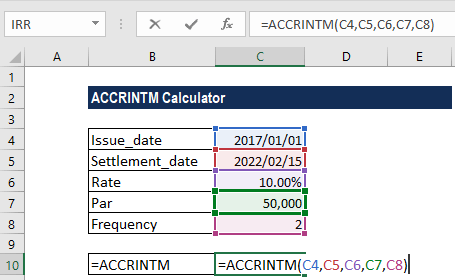We get the result below: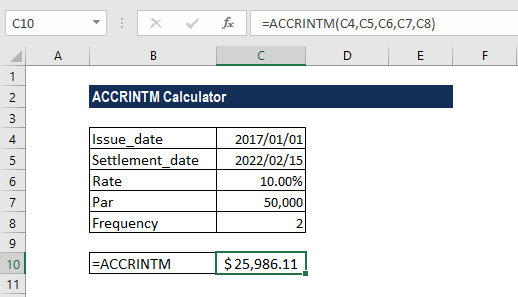#### Example 2 – Using DATE with ACCRINT

Let’s now see how we can use the DATE function along with ACCRINTM to find out the interest payable. The reason we use the DATE function is that the dates given are not in date format. We can use DATE along with ACCRINT to get the interest receivable on maturity.

Suppose we are given the following data: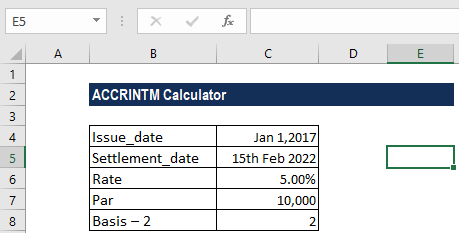The formula to use is shown below: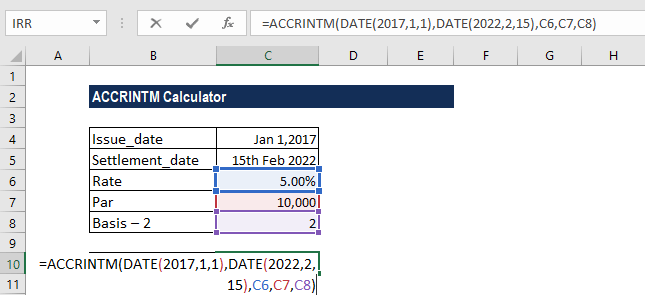We get the result below: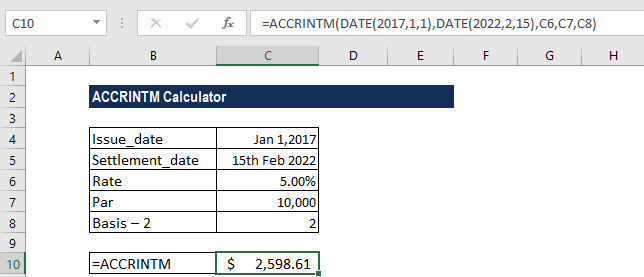#### Example 3

Let’s now see what happens when do not provide par value. Suppose we are given the following data: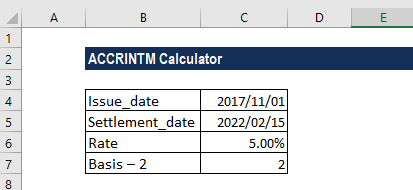There is no par value given. The formula to use is shown below: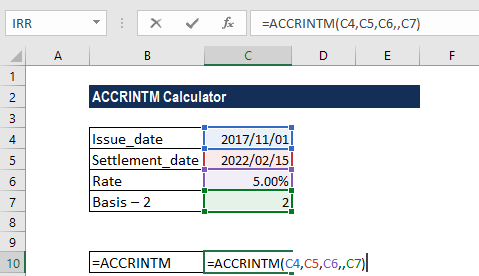The ACCRINTM function took the par value as \$1,000, which is the default value. The result we got was for the interest calculation at a par value of \$1,000: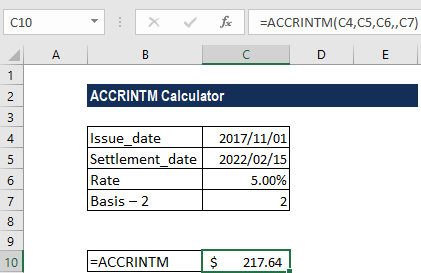### Things to remember about the ACCRINTM Function

1. #NUM! error – Occurs when:
• The given rate argument is ≤ 0 or the given [par] argument is ≤ 0.
• The provided issue argument is greater than or equal to the settlement date. For example, when we provide =ACCRINTM(“1/10/2017″,”1/7/2017”,0.05,,1).
• The given basis argument is not equal to 0, 1, 2, 3, or 4. For example, when we provide =ACCRINTM(“1/1/2017″,”1/7/2017”,0.05,10000,5).
1. #VALUE! error – Occurs when:
• The given issue or settlement arguments are not valid dates.
• Any of the arguments provided is non-numeric.
1. When we input the issue and settlement dates, they should be entered as either:
• References to cells that contain dates; or
• Dates that are returned from formulas; or
• If we attempt to input the date arguments as text, MS Excel may interpret them incorrectly, due to different date systems or date interpretation settings.

Thanks for reading CFI’s guide to important Excel functions! By taking the time to learn and master these functions, you’ll significantly speed up your financial analysis. To learn more, check out these additional CFI resources:

• Excel Functions for Finance